# StretchMin, StretchMaxHow do I stop a table column stretching so far that it is mostly white space?

How do I stop a table column shrinking too much so that I can’t read anything?

If I was responsible for an organisations code base and coding standards, with the release of Genero Enterprise 4.00 and the various responsive features, one of the coding standards I would add is that table columns that a) contain character data and b) have a width greater than 15, I would say that these columns should have STRETCH=X.  Most codes and numeric values are consistent in their width and less than 15 characters, whilst most name, description, address type data are textual, vary in length and are often designed with a maximum length that is rarely encountered.  By adding STRETCH=X to these columns, they will stretch and shrink in width in response to the width of the table.

I can show this with this example, the code for which is at the end of the article. The first series of screenshots show what happens without STRETCH=X and the parent window is resized smaller and bigger.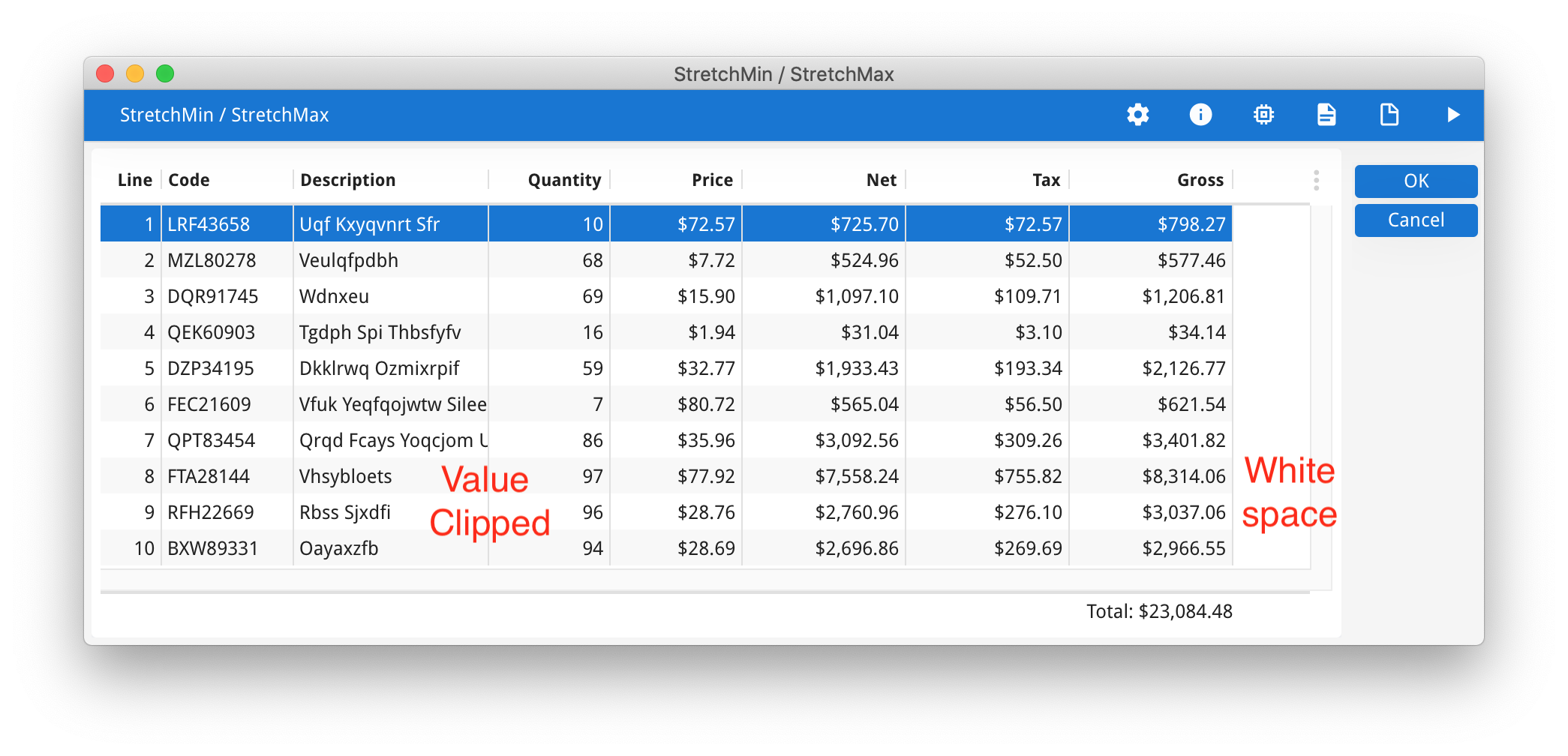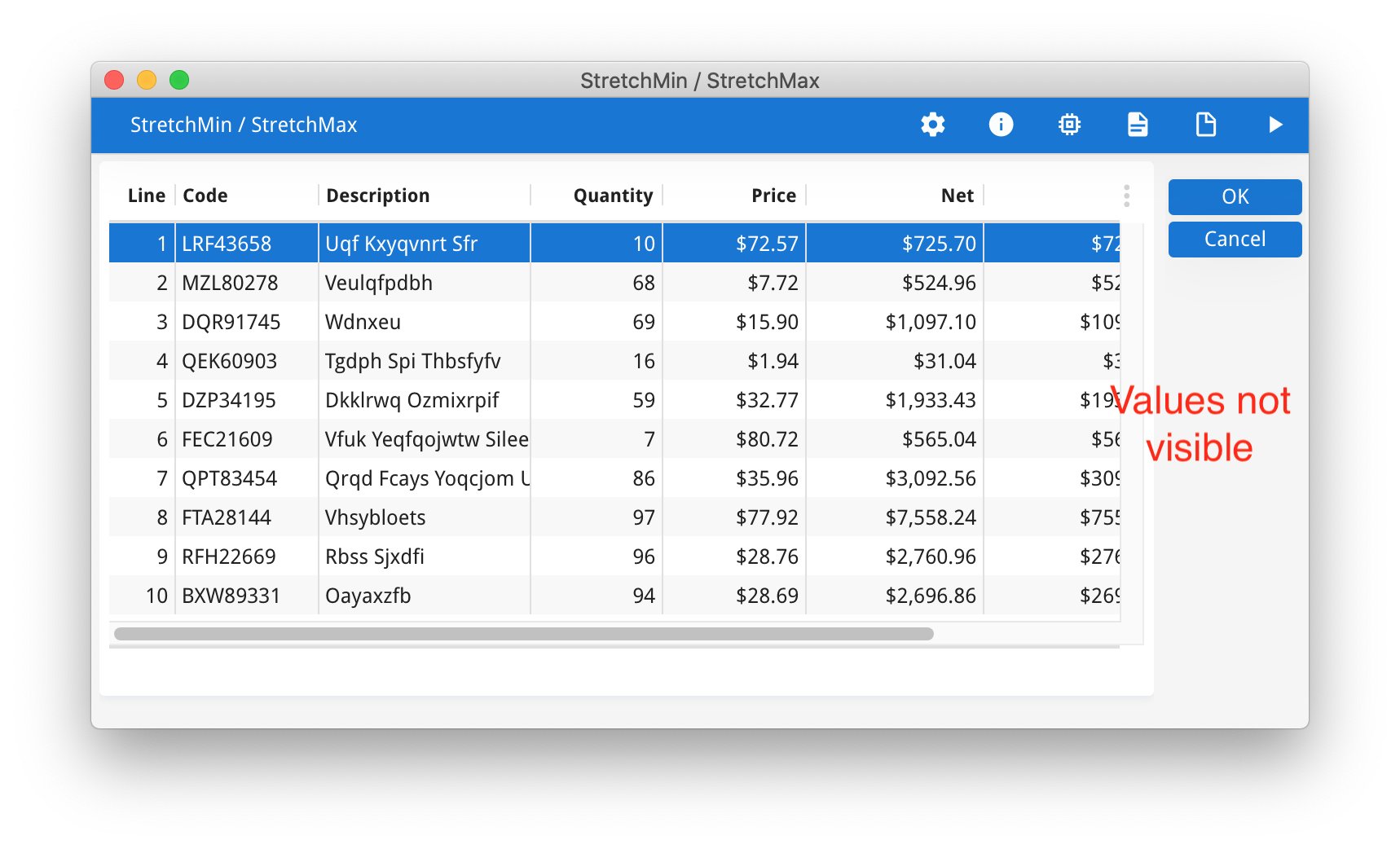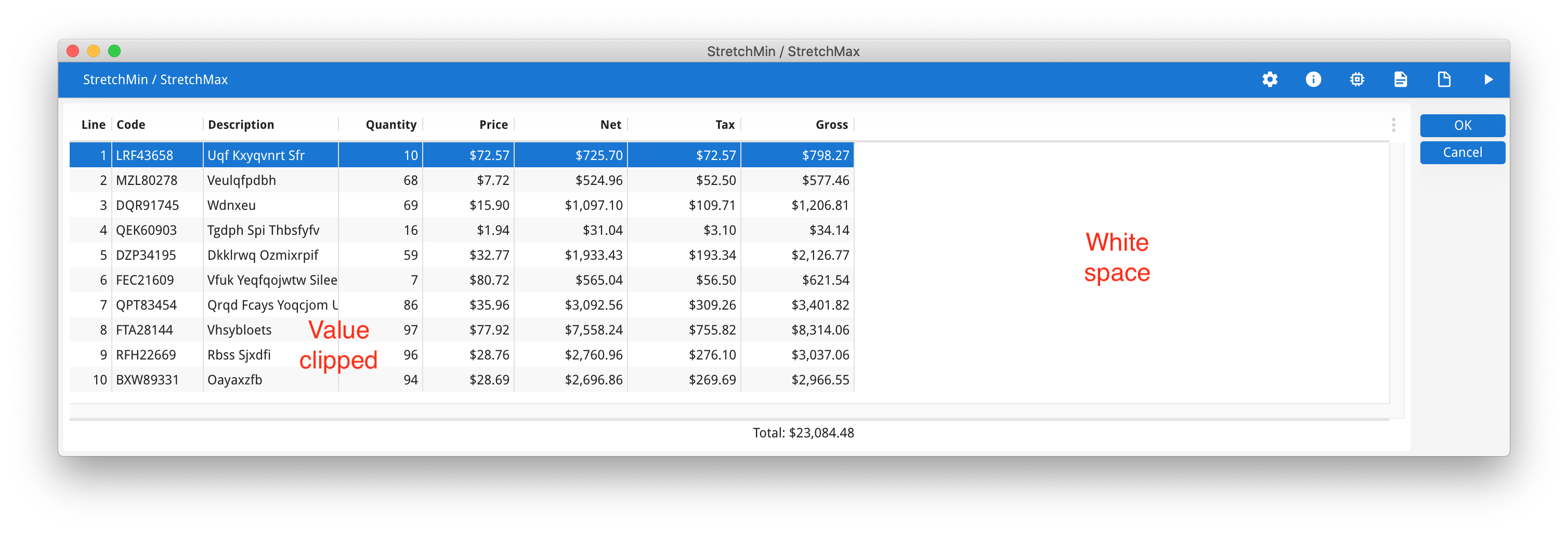Note how when the table is smaller, some data is obscured including the Grand Total, but there is unused space in the description column.  Note how when the table is wider, there is some white space to the right.

These next three screenshots, I have added STRETCH=X to the Description column.  Note how in response to the table getting bigger or smaller, the description column shrinks or grows.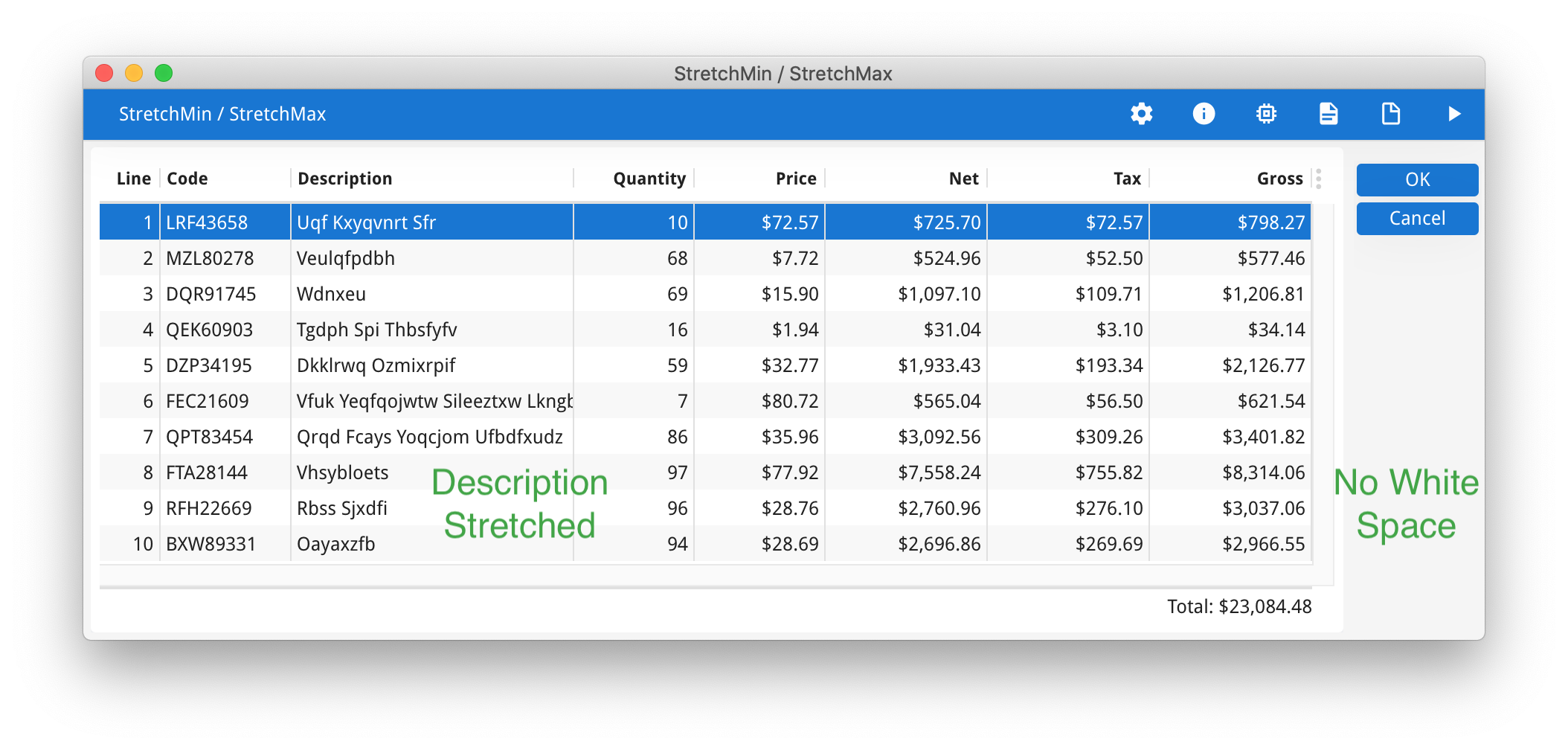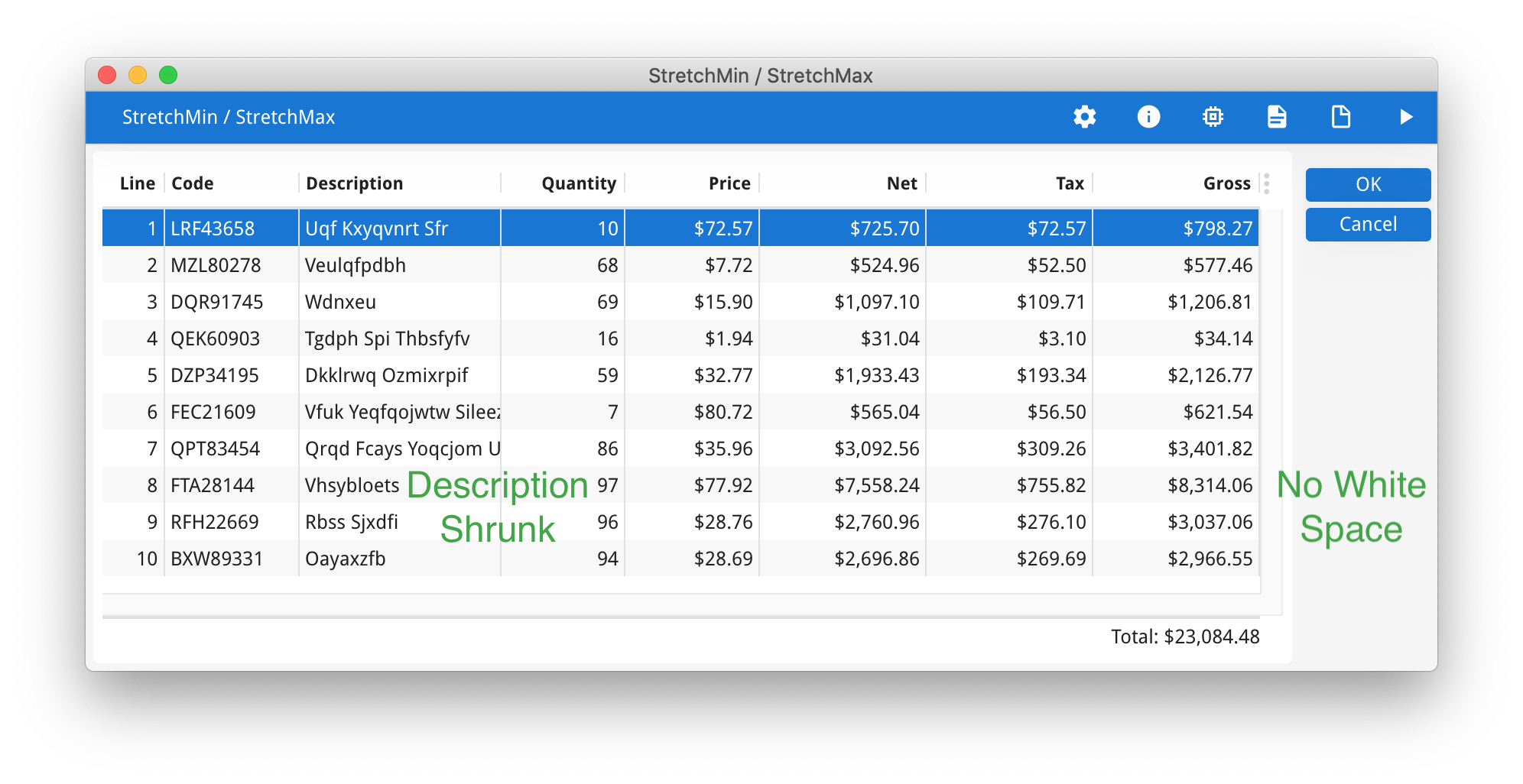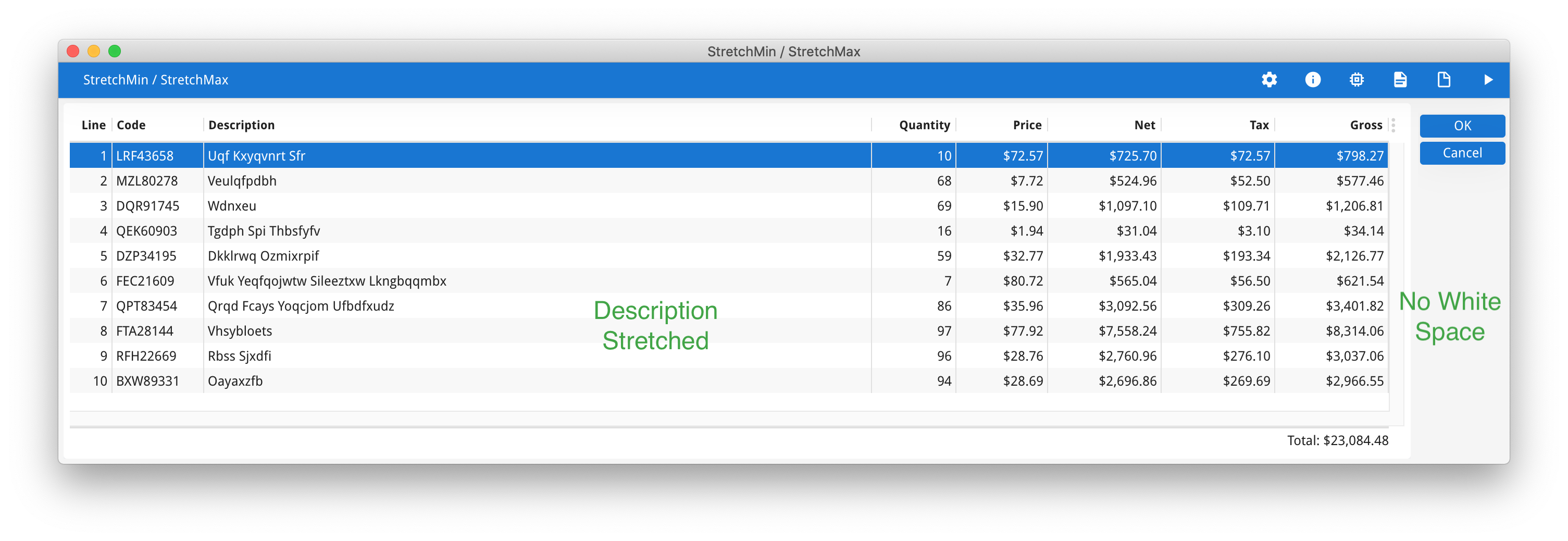There is no white space to the right and there is less chance of important data being obscured.

The minimum width that a column will shrink to is the number of cells used by the layout tag in the form design.  There is no default maximum constraint as to how wide a column will stretch.

The new STRETCHMIN and STRETCHMAX attributes allow you to override this minimum and maximum width that a column can shrink and grow to.

A column (for example a name or description) might have a width of 15 defined by the layout tag in the form definition. You can use STRETCHMIN to change that value, you might decide to set it so that a column can shrink further but always make sure the column title is always visible.  In this example, note how I shrunk the window, the description column has shrunk also but only so far that the title “Description” is still completely visible.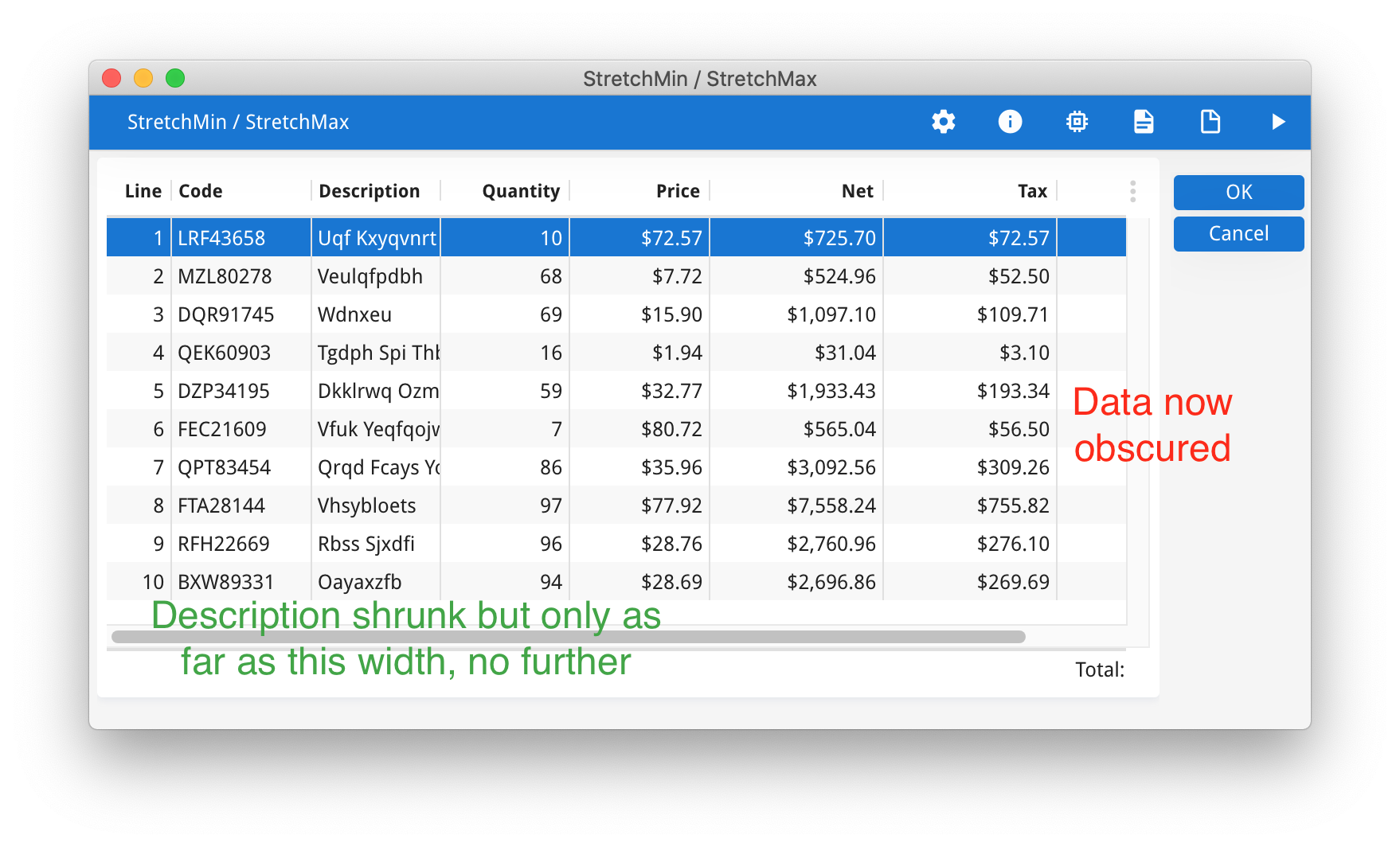As the name implies, STRETCHMAX applies a maximum width to the column.  This will prevent the case that there is too much white space inside the Table.  In this next screenshot, it is better to have some whitespace to the right rather than in the Description column between the numeric data and the labels for each row of data.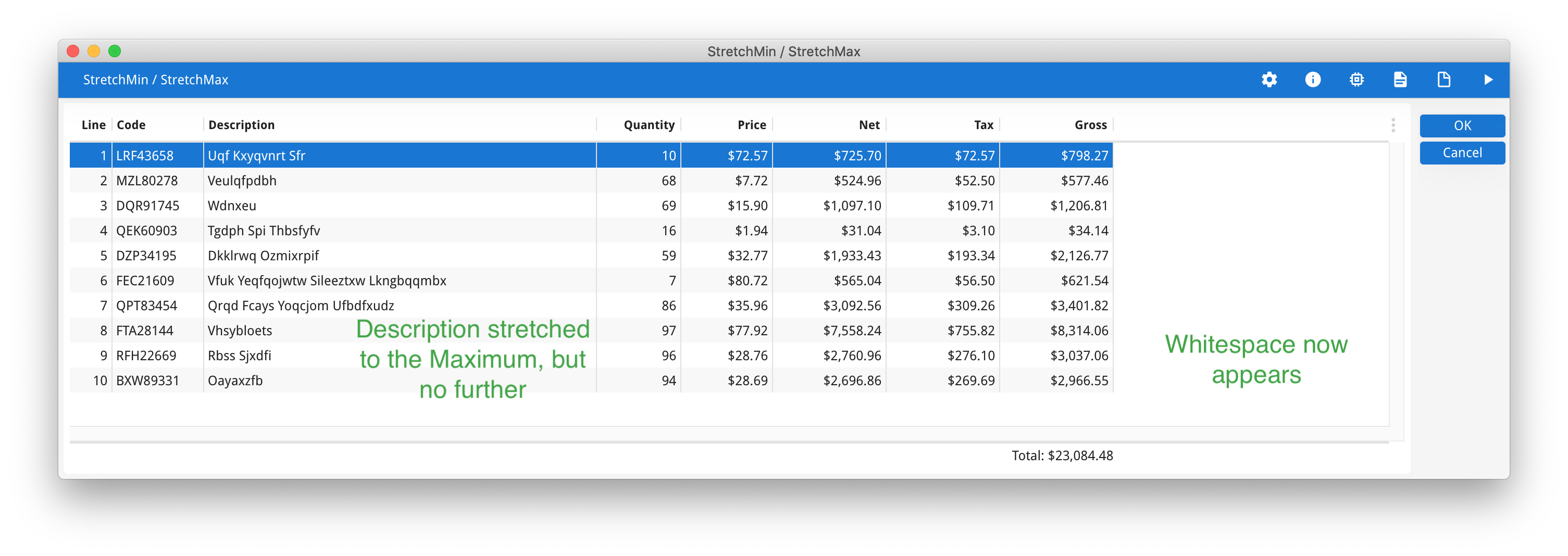• STRETCHMIN, STRETCHMAX can be specified using the media size selector @ e.g. STRETCHMIN@SMALL=5, STRETCHMIN@MEDIUM=10.  This enables you to target the stretch characteristics for different devices.
• Due to proportional fonts, the units for STRETCHMIN, STRETCHMAX do not equate to the number of characters.  Note that I did not need to set STRETCHMIN=12 in order to display the 12 characters in “Description”
• When there are multiple columns that have STRETCH=X, they will each stretch and shrink in proportion.
• The STRETCHMIN, STRETCHMAX currently only apply inside a TABLE container, they do not apply inside a GRID or SCROLLGRID

As I said at the start, I would consider adding STRETCH=X to certain columns as part of my coding standards.  As part of that you may wish to also have standards about the use of STRETCHMIN, and STRETCHMAX.  Perhaps STRETCHMIN set to that the column title is at a minimum visible, and STRETCHMAX set to a factor of the maximum number of characters that can be displayed to prevent excessive white space.

The code for the above screenshots is below.  Note the comments in the form about the application of STRETCH and STRETCHMIN, STRETCHMAX to the description column.

```#! stretchmin.per
LAYOUT (TEXT="StretchMin / StretchMax")
TABLE
{
[f00|f01     |f02           |f03    |f04     |f05        |f06        |f07        ]
[a07        ]
}
END
END
ATTRIBUTES
f00 = formonly.line, TITLE="Line";
f01 = formonly.code, TITLE="Code";

-- Uncomment one of these next three lines at a time
f02 = formonly.desc, TITLE="Description", SCROLL;  -- No stretch
--f02 = formonly.desc, TITLE="Description", SCROLL, STRETCH=X;
--f02 = formonly.desc, TITLE="Description", SCROLL, STRETCH=X, STRETCHMIN=8, STRETCHMAX=50;

f03 = formonly.quantity, TITLE="Quantity", FORMAT="---,--&";
f04 = formonly.price, TITLE="Price", FORMAT="--\$&.&&";
f05 = formonly.net, TITLE="Net", FORMAT="----,-\$&.&&";
f06 = formonly.tax, TITLE="Tax", FORMAT="----,-\$&.&&";
f07 = formonly.gross, TITLE="Gross", FORMAT="----,-\$&.&&";
AGGREGATE a07 = FORMONLY.gross_total, AGGREGATETEXT = "Total:", AGGREGATETYPE = SUM;

INSTRUCTIONS
SCREEN RECORD scr(line,code, desc, quantity, price, net, tax, gross)```

```#! stretchmin.4gl
IMPORT util
TYPE line_type RECORD
idx INTEGER,
code CHAR(10),
desc STRING,
quantity DECIMAL(11, 2),
price DECIMAL(11, 2),
net DECIMAL(11, 2),
tax DECIMAL(11, 2),
gross DECIMAL(11, 2)
END RECORD
DEFINE arr DYNAMIC ARRAY OF line_type

MAIN

CLOSE WINDOW SCREEN

CALL populate()
OPEN WINDOW w WITH FORM "stretchmin_table"
DISPLAY ARRAY arr TO scr.*
END MAIN

FUNCTION populate()
DEFINE i INTEGER

FOR i = 1 TO 10
LET arr[i].idx = i
LET arr[i].code = random_code()
LET arr[i].desc = random_text()
LET arr[i].quantity = util.Math.rand(100) + 1
LET arr[i].price = (100 + util.Math.rand(10000)) / 100
LET arr[i].net = arr[i].quantity * arr[i].price
LET arr[i].tax = arr[i].net * 0.1
LET arr[i].gross = arr[i].net + arr[i].tax
END FOR

END FUNCTION

FUNCTION random_code()
DEFINE sb base.StringBuffer
DEFINE i INTEGER

LET sb = base.StringBuffer.create()

FOR i = 1 TO 3
CALL sb.append(ASCII (util.Math.rand(26) + 65))
END FOR
CALL sb.append(util.Math.rand(100000) USING "&&&&&")
RETURN sb.toString()
END FUNCTION

FUNCTION random_text()
DEFINE
word_count INTEGER,
letter_count INTEGER
DEFINE
word_idx INTEGER,
letter_idx INTEGER
DEFINE sb base.StringBuffer

LET sb = base.StringBuffer.create()
LET word_count = util.Math.rand(4) + 1
FOR word_idx = 1 TO word_count
IF word_idx > 1 THEN
CALL sb.append(" ")
END IF
LET letter_count = util.Math.rand(8) + 3
FOR letter_idx = 1 TO letter_count
IF letter_idx = 1 THEN
CALL sb.append(ASCII (util.Math.rand(26) + 65))
ELSE
CALL sb.append(ASCII (util.Math.rand(26) + 97))
END IF
END FOR
END FOR
RETURN sb.toString()

END FUNCTION```

```#! stretchmin.4st
<?xml version="1.0" encoding="ANSI_X3.4-1968"?>
<StyleList>
<Style name="Window">
<StyleAttribute name="windowType" value="normal" />
</Style>
<Style name="Table">